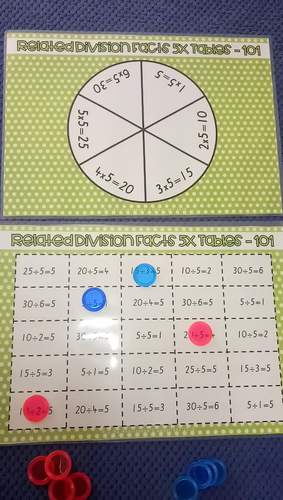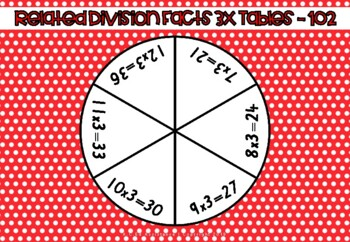# Related Division Facts Spinner Games - 2, 3, 5 and 10 Times Tables

Rated 5 out of 5, based on 2 reviews
2 Ratings;
3rd - 5th
Subjects
Resource Type
Standards
Formats Included
• PDF
Pages
21 pages
Report this resource to TPT

### Description

Numeracy

These SPINNER GAMES have been leveled and are ideal for differentiation in your class to cater for mixed-ability groups, as well as ensuring that learners at different starting points can receive the instruction they need to GROW and SUCCEED!

- The first level (board 101) covers 1 times... through to 6 times...

- The second level (board 102) covers 7 times... through to 12 times...

These games help students explore the relationship between multiplication and division, so they can fluently perform these operations. Since related division facts are part of the National Curriculum, learning multiplication tables and inverse operations are important foundations for learning different aspects of mathematics such as: division, algebra, long multiplication, and even fractions!

Australian Curriculum: Numeracy Year 3

Recall multiplication facts of two, three, five and ten and related division facts (ACMNA056)

Head on over to my Instagram account @_miss_carlee_ and follow me to see regular classroom inspo, resources and teacher wellbeing!

A QUICK TIP FOR YOU...

Did you know that you can earn TpT credits to use for future purchases? Go to your 'My Purchases' page and next to each product that you've already purchased, you will notice a 'Provide Feedback' button. Click on it and take a minute to provide some positive feedback! Every time you do this, TpT rewards you with credits, which you can use as a deduction toward future purchases!

I highly value your feedback and comments. It not only warms my heart, but it helps me to determine which resources are most valuable for teachers. I always look forward to creating new products and supporting you in the classroom!

Total Pages
21 pages
N/A
Teaching Duration
N/A
Report this resource to TPT
Reported resources will be reviewed by our team. Report this resource to let us know if this resource violates TPT’s content guidelines.

### Standards

to see state-specific standards (only available in the US).
Apply properties of operations as strategies to multiply and divide. Examples: If 6 × 4 = 24 is known, then 4 × 6 = 24 is also known. (Commutative property of multiplication.) 3 × 5 × 2 can be found by 3 × 5 = 15, then 15 × 2 = 30, or by 5 × 2 = 10, then 3 × 10 = 30. (Associative property of multiplication.) Knowing that 8 × 5 = 40 and 8 × 2 = 16, one can find 8 × 7 as 8 × (5 + 2) = (8 × 5) + (8 × 2) = 40 + 16 = 56. (Distributive property.)
Understand division as an unknown-factor problem. For example, find 32 ÷ 8 by finding the number that makes 32 when multiplied by 8.
Fluently multiply and divide within 100, using strategies such as the relationship between multiplication and division (e.g., knowing that 8 × 5 = 40, one knows 40 ÷ 5 = 8) or properties of operations. By the end of Grade 3, know from memory all products of two one-digit numbers.
Identify arithmetic patterns (including patterns in the addition table or multiplication table), and explain them using properties of operations. For example, observe that 4 times a number is always even, and explain why 4 times a number can be decomposed into two equal addends.